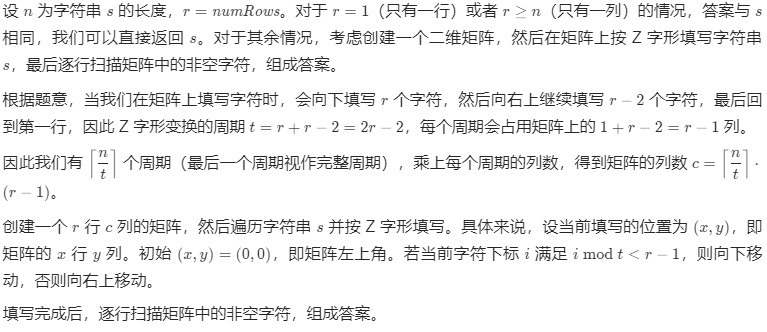• 累计撰写 184 篇文章
• 累计创建 20 个标签
• 累计收到 2 条评论

### 目 录CONTENT# JAVA经典题库：6. Z 字形变换（难度：中等）2022-11-04 / 0 评论 / 0 点赞 / 250 阅读 / 1,304 字 / 正在检测是否收录...

# 题目

P   A   H   N
A P L S I I G
Y   I   R


string convert(string s, int numRows);






输入：s = "PAYPALISHIRING", numRows = 4

P     I    N
A   L S  I G
Y A   H R
P     I


输入：s = "A", numRows = 1



1 <= s.length <= 1000
s 由英文字母（小写和大写）、',' 和 '.' 组成
1 <= numRows <= 1000


# 文字题解

## 方法一：利用二维矩阵模拟class Solution {
public String convert(String s, int numRows) {
int n = s.length(), r = numRows;
if (r == 1 || r >= n) {
return s;
}
int t = r * 2 - 2;
int c = (n + t - 1) / t * (r - 1);
char[][] mat = new char[r][c];
for (int i = 0, x = 0, y = 0; i < n; ++i) {
mat[x][y] = s.charAt(i);
if (i % t < r - 1) {
++x; // 向下移动
} else {
--x;
++y; // 向右上移动
}
}
StringBuffer ans = new StringBuffer();
for (char[] row : mat) {
for (char ch : row) {
if (ch != 0) {
ans.append(ch);
}
}
}
return ans.toString();
}
}



• 时间复杂度：O(r⋅n)，其中 r=numRows，n 为字符串 s 的长度。时间主要消耗在矩阵的创建和遍历上，矩阵的行数为 r，列数可以视为 O(n)。

• 空间复杂度：O(r⋅n)。矩阵需要 O(r⋅n) 的空间。

## 方法二：压缩矩阵空间

class Solution {
public String convert(String s, int numRows) {
int n = s.length(), r = numRows;
if (r == 1 || r >= n) {
return s;
}
StringBuffer[] mat = new StringBuffer[r];
for (int i = 0; i < r; ++i) {
mat[i] = new StringBuffer();
}
for (int i = 0, x = 0, t = r * 2 - 2; i < n; ++i) {
mat[x].append(s.charAt(i));
if (i % t < r - 1) {
++x;
} else {
--x;
}
}
StringBuffer ans = new StringBuffer();
for (StringBuffer row : mat) {
ans.append(row);
}
return ans.toString();
}
}



• 时间复杂度：O(n)。

• 空间复杂度：O(n)。压缩后的矩阵需要 O(n)的空间。

## 方法三：直接构造

Python3：

class Solution:
def convert(self, s: str, numRows: int) -> str:
n, r = len(s), numRows
if r == 1 or r >= n:
return s
t = r * 2 - 2
ans = []
for i in range(r):  # 枚举矩阵的行
for j in range(0, n - i, t):  # 枚举每个周期的起始下标
ans.append(s[j + i])  # 当前周期的第一个字符
if 0 < i < r - 1 and j + t - i < n:
ans.append(s[j + t - i])  # 当前周期的第二个字符
return ''.join(ans)



Golang：


func convert(s string, numRows int) string {
n, r := len(s), numRows
if r == 1 || r >= n {
return s
}
t := r*2 - 2
ans := make([]byte, 0, n)
for i := 0; i < r; i++ { // 枚举矩阵的行
for j := 0; j+i < n; j += t { // 枚举每个周期的起始下标
ans = append(ans, s[j+i]) // 当前周期的第一个字符
if 0 < i && i < r-1 && j+t-i < n {
ans = append(ans, s[j+t-i]) // 当前周期的第二个字符
}
}
}
return string(ans)
}


c++：

class Solution {
public:
string convert(string s, int numRows) {
int n = s.length(), r = numRows;
if (r == 1 || r >= n) {
return s;
}
string ans;
int t = r * 2 - 2;
for (int i = 0; i < r; ++i) { // 枚举矩阵的行
for (int j = 0; j + i < n; j += t) { // 枚举每个周期的起始下标
ans += s[j + i]; // 当前周期的第一个字符
if (0 < i && i < r - 1 && j + t - i < n) {
ans += s[j + t - i]; // 当前周期的第二个字符
}
}
}
return ans;
}
};


• 时间复杂度：O(n)，其中 n 为字符串 s 的长度。s 中的每个字符仅会被访问一次，因此时间复杂度为 O(n)。
• 空间复杂度：O(1)。返回值不计入空间复杂度。
0